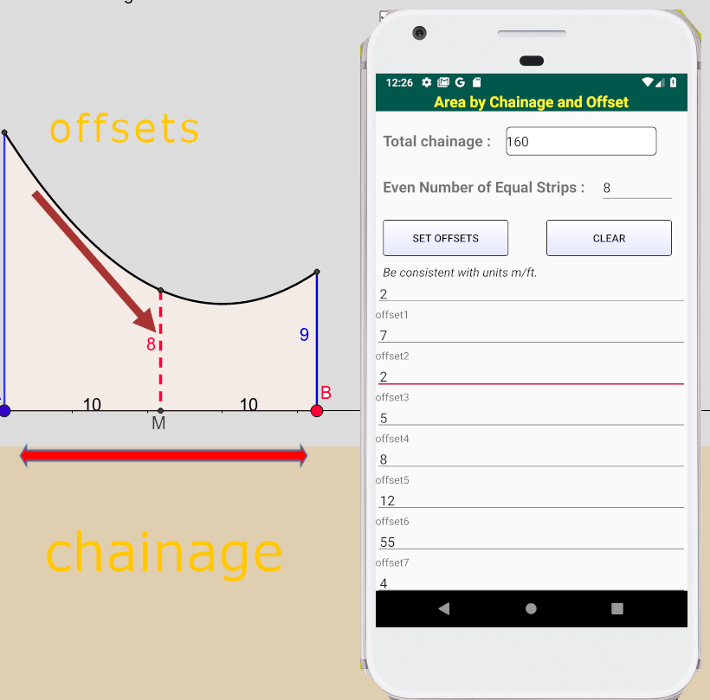## Area byChainage and Offset

### Steps used Simpsons rule

Simpson's Rule. It states that, sum of first and last ordinates has to be done. Add twice the sum of remaining odd ordinates and four times the sum of remaining even ordinates. Multiply to this total sum by 1/3rd of the common distance between the ordinates which gives the required area.

• The area has to be divided into an even number of parallel strips perpendicular to a base line(the length is the total chainage)
• With an even number of strips we will have an odd number of offsets bounding the area vertically. Enter this information and generate input boxes for the offset values.
• Measure these offsets and and enter them into the input textboxes. Press calculate to compute the area of the irregular shape.For regular shapes:

Circles  - pi*r2

Triangles

Learn the Triangle Inequality Theorem. This theorem simply states that the sum of two sides of a triangle must be greater than the third side. If this is true for all three combinations, then you will have a valid triangle. You'll have to go through these combinations one by one to make sure that the triangle is possible. You can also think of the triangle as having the side lengths a, b, and c and the theorem being an inequality, which states: a+b > c, a+c > b, and b+c > a.

The app will do these checks for you.

Herons formula is used: https://www.mathopenref.com/heronsformula.html